##全国校区# 教你用Python批量实现文件夹下所有Excel文件的第二张表合并2022-01-27

1、将A文件中名为a的sheet和B文件中名为b的sheet合并到一个sheet中去。

2、将文件夹下所有文件的第二张表合并。我做出来了，核心部分没有用pandas，而且逻辑比较繁琐。想求一用pandas解决的简洁方案。

# 2、解决方法

```# coding: utf-8 # 将A文件中名为a的sheet和B文件中名为b的sheet合并到一个sheet中去 from pathlib import Path import pandas as pd

path = r'E:PythonCrawler有趣的代码Python自动化办公将A文件中名为a的sheet和B文件中名为b的sheet合并到一个sheet中去' data_ex1 = pd.read_excel('ex1.xlsx', sheet_name='df1')
result = pd.concat([data_ex1, data_ex2], ignore_index=True)
result.to_excel('将A文件中名为a的sheet和B文件中名为b的sheet合并到一个sheet中去.xlsx', index=False, encoding='utf-8')
print('添加和合并完成！')```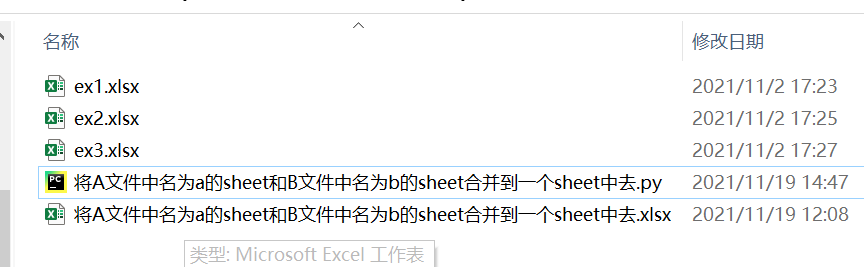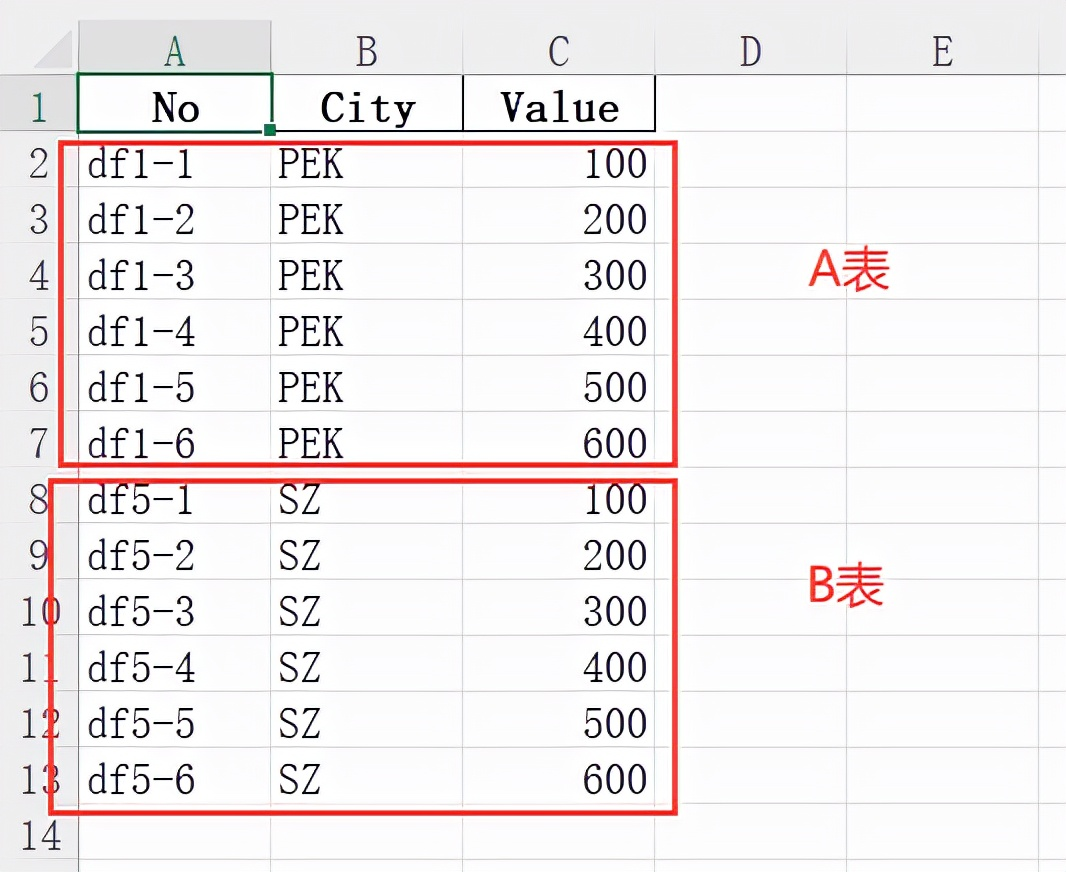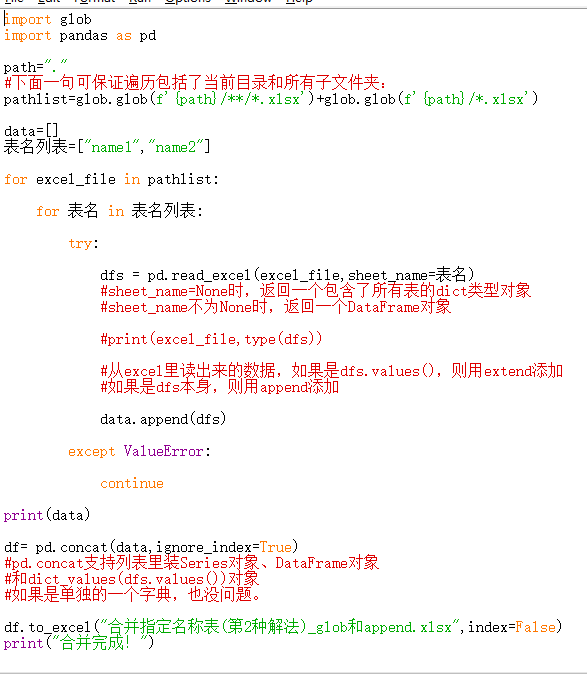```# coding: utf-8 # 合并所有表格中的第二张表格 from pathlib import Path import pandas as pd

path = Path(r'E:PythonCrawler有趣的代码Python自动化办公将文件夹下所有文件的第二张表合并')
data_list = [] for i in path.glob("*.xls*"): # data = pd.read_excel(i, sheet_name='df2') data = pd.read_excel(i, sheet_name=1)
data_list.append(data)

result = pd.concat(data_list, ignore_index=True)
result.to_excel(path.joinpath('取所有excel表的df2表进行合并.xlsx'), index=False, encoding='utf-8')
print('添加和合并完成！')```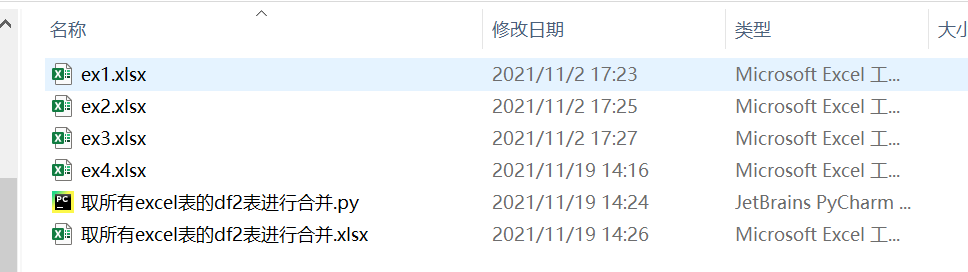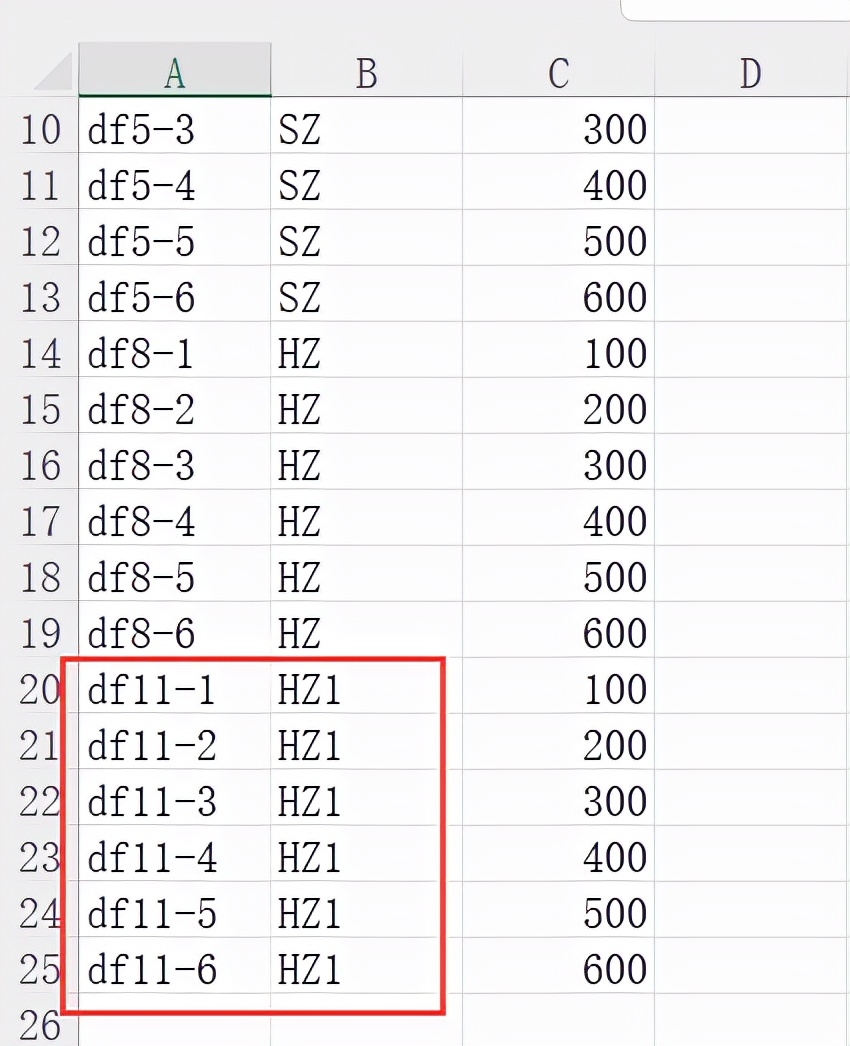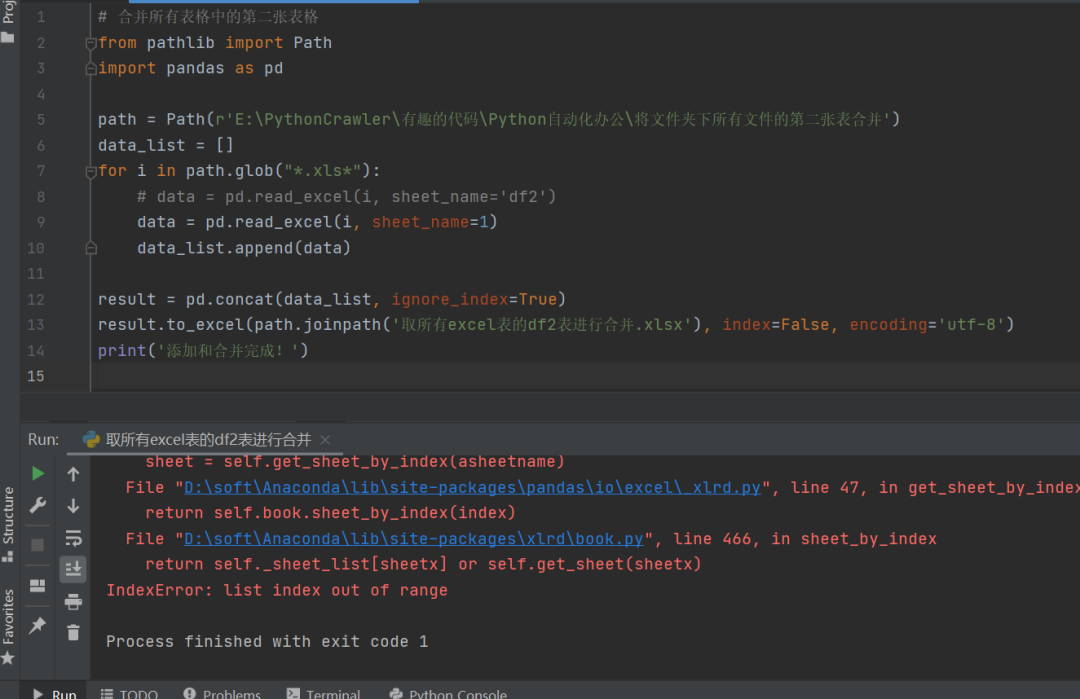# 3、总结

OK